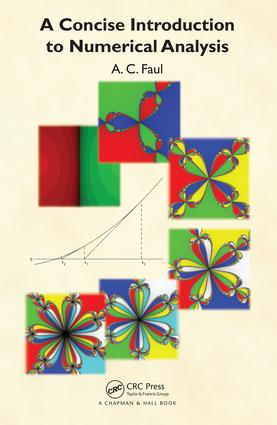A Concise Introduction to Numerical Analysis

1st Edition

Chapman and Hall/CRC

292 pages | 49 B/W Illus.

Purchasing Options:\$ = USD
Hardback: 9781498712187
pub: 2016-03-15
SAVE ~\$17.19
\$85.95
\$68.76
x
eBook (VitalSource) : 9781315370217
pub: 2018-10-24
from \$42.98

FREE Standard Shipping!

Description

This textbook provides an accessible and concise introduction to numerical analysis for upper undergraduate and beginning graduate students from various backgrounds. It was developed from the lecture notes of four successful courses on numerical analysis taught within the MPhil of Scientific Computing at the University of Cambridge. The book is easily accessible, even to those with limited knowledge of mathematics.

Students will get a concise, but thorough introduction to numerical analysis. In addition the algorithmic principles are emphasized to encourage a deeper understanding of why an algorithm is suitable, and sometimes unsuitable, for a particular problem.

A Concise Introduction to Numerical Analysis strikes a balance between being mathematically comprehensive, but not overwhelming with mathematical detail. In some places where further detail was felt to be out of scope of the book, the reader is referred to further reading.

The book uses MATLAB® implementations to demonstrate the workings of the method and thus MATLAB's own implementations are avoided, unless they are used as building blocks of an algorithm. In some cases the listings are printed in the book, but all are available online on the book’s page at www.crcpress.com.

Most implementations are in the form of functions returning the outcome of the algorithm. Also, examples for the use of the functions are given. Exercises are included in line with the text where appropriate, and each chapter ends with a selection of revision exercises. Solutions to odd-numbered exercises are also provided on the book’s page at www.crcpress.com.

This textbook is also an ideal resource for graduate students coming from other subjects who will use numerical techniques extensively in their graduate studies.

Reviews

"This is a thorough and detailed introduction to the important topic of numerical analysis. It is based upon an existing successful postgraduate course and assumes a significant level of mathematical sophistication. The material is very clearly presented, with lots of end-of-topic questions and examples in MATLAB that really illustrate the key issues involved, such as error propagation. The range of topics is conventional, but the level of detail is not. This is definitely a textbook that should be on the shelf of anyone working in the field who wants a deep understanding of the methods, limitations, and practical issues."

—Dr. Matt Probert, Department of Physics, University of York, UK

Fundamentals

Floating Point Arithmetic

Overflow and Underflow

Absolute, Relative Error, Machine Epsilon

Forward and Backward Error Analysis

Loss of Significance

Robustness

Error Testing and Order of Convergence

Computational Complexity

Condition

Revision Exercises

Linear Systems

Simultaneous Linear Equations

Gaussian Elimination and Pivoting

LU Factorization

Cholesky Factorization

QR Factorization

The Gram–Schmidt Algorithm

Givens Rotations

Householder Reflections

Linear Least Squares

Singular Value Decomposition

Iterative Schemes and Splitting

Jacobi and Gauss–Seidel Iterations

Relaxation

Steepest Descent Method

Krylov Subspaces and Pre-Conditioning

Eigenvalues and Eigenvectors

The Power Method

Inverse Iteration

Deflation

Revision Exercises

Interpolation and Approximation Theory

Lagrange Form of Polynomial Interpolation

Newton Form of Polynomial Interpolation

Polynomial Best Approximations

Orthogonal polynomials

Least-Squares Polynomial Fitting

The Peano Kernel Theorem

Splines

B-Spline

Revision Exercises

Non-Linear Systems

Bisection, Regula Falsi, and Secant Method

Newton’s Method

Broyden’s Method

Householder Methods

Müller’s Method

Fixed Point Iteration Theory

Mixed Methods

Revision Exercises

Numerical Integration

Mid-Point and Trapezium Rule

The Peano Kernel Theorem

Simpson’s Rule

Newton–Cotes Rules

Composite Rules

Multi-Dimensional Integration

Monte Carlo Methods

Revision Exercises

ODEs

One-Step Methods

Multistep Methods, Order, and Consistency

Order Conditions

Stiffness and A-Stability

Backward Differentiation Formulae

The Milne and Zadunaisky Device

Rational Methods

Runge–Kutta Methods

Revision Exercises

Numerical Differentiation

Finite Differences

Differentiation of Incomplete or Inexact Data

PDEs

Classification of PDEs

Parabolic PDEs

Elliptic PDEs

Parabolic PDEs in Two Dimensions

Hyperbolic PDEs

Spectral Methods

Finite Element Method

Revision Exercises

Anita C Faul

Cambridge, Cambridgeshire, United Kingdom